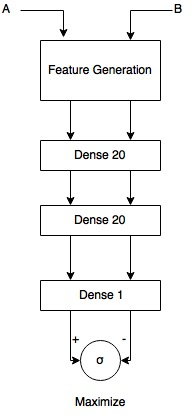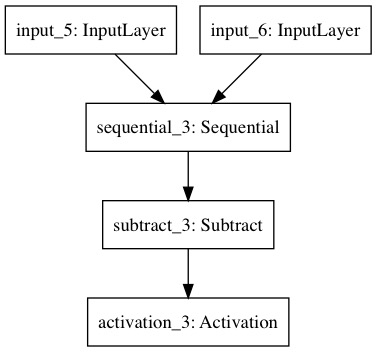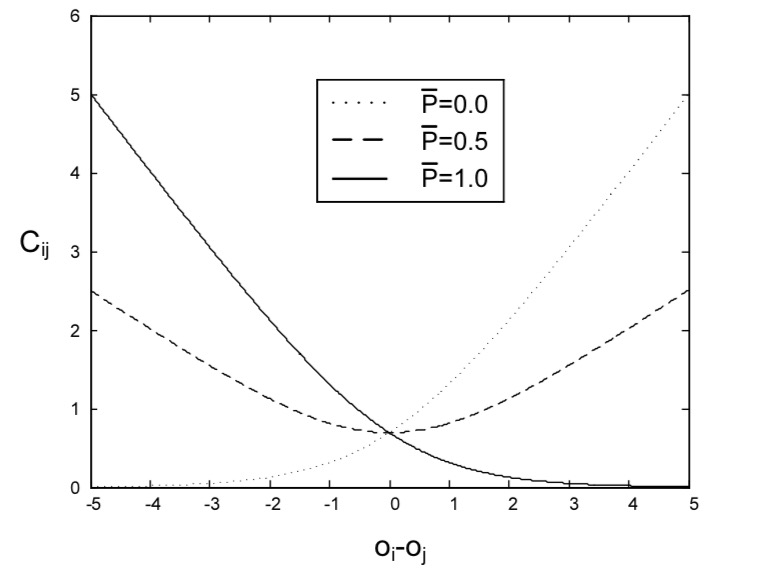# Learning to Rank w/ Siamese Networks & Pairwise data

Alex Egg,

Given a set of items, there is value in being able to rank them from best to worst. A common way to do this is to have a scoring function and then pasing the items in the set through the scoring function, then sorting the scores to give an overall rank. There is a way to learn the scoring function by using pairwise data: in this scenario, we take a pair where one item is higher ranked than the other and then we pass it through a scoring function and try to maximize the difference between the two scores. If we are able to consitently differentiate between the pairs, then we will have been able to learn a scoring function.

I was originally exposed to this technique in the Learning to rank  paper by Burges. This paper uses pair-wise data and a Siamese Archecture to learn a scoring function.

For the full code see the github repo: https://github.com/eggie5/RankNet/blob/master/RankNet.ipynb

## ArchectureFigure 1: Siamese Nework - Scores between pairs are maximized using binary cross-entropy

### Base Network/Scoring Function

This is scoring function is a regression which takes an embedding (some vector describing the item to be scored/ranked) and outputs a single neuron: the score of the item. The network is composed of two of these, one for each item in the pair.

In Figure 1 above, the base network is a Deep CNN w/ the traditional softmax removed from the end and replaced w/ a single neuron. The inputs A & B are of course images.

def create_base_network(input_dim):
seq = Sequential()
return seq


### Meta Network

The Meta Network takes the outputs of the base network and takes the difference. The cost function then returns a proporital positive on how big the differnece is between the two pairs and a negative feedback if the difference is negative (meaning the ranking was incorrect).

def create_meta_network(input_dim, base_network):
input_a = Input(shape=(input_dim,))
input_b = Input(shape=(input_dim,))

rel_score = base_network(input_a)
irr_score = base_network(input_b)

# subtract scores
diff = Subtract()([rel_score, irr_score])

# Pass difference through sigmoid function.
prob = Activation("sigmoid")(diff)

# Build model.
model = Model(inputs = [input_a, input_b], outputs = prob)
model.compile(optimizer = "adam", loss = "binary_crossentropy")

return modelFigure 2: Siamese Network diagram - The Sequential block in the diagram is any type of function which takes the input vector and outputs a scalar. In this example it’s a Neural Network which is learnt. We then subtract the two scores from the pair and pass that to the Activation Sigmoid which is then used in the cost function.

### Data & Cost Function

We can use the binary cross-entropy cost function w/ the pairwise data. The label for an instance is a number between 1-0 where 0 means that the right item is 100% more important .5 means the pair is equal and 1 means the left item is 100% more important. The binary cross entropy then compare the sigmoid of the predicted score (probability) w/ the actual probability which then gives feedback to the gradient descent backprop routine.

where $o_{ij}$ is the difference of the output from the scoring function for both items of the pair $i$ and $j$.Figure 3: Example values of the cost function for 3 different class labels. For example if the training example is a 1, this means the left item is higher ranked. Therefore $C_{ij}$ is very small when the difference is positive large and vary large when the difference is negative giving feedback to the backprop.

## Case Study: Ranking Search Results

This was the original use-case explored in the paper: how to more effectivly sort search results. They looked at past examples of click stream data and quries and learnt a scoring function to help sort the results.

## Case Study: Image Selection

A common use-case for these types of networks is to automate the selection of an image from a set of images in order to choose the best one by some criteria. The training data would be annotated pairs where the labels would be which image is better according to the criteria. For example, the best quality image. Expedia and trip advisor did something like this to help automate and scale up the default image selection process of a hotel’s landing page. For example, show a nice picture of the view or room then the toilet as the first image the user sees.

 Burges, Chris, et al. “Learning to rank using gradient descent.” Proceedings of the 22nd international conference on Machine learning. ACM, 2005.